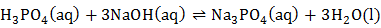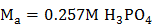### Sample Problem

A 17.5 mL sample of phosphoric acid was titrated with 45.0 mL of 0.300 M NaOH solution. What is the concentration of the sample given the acid-base reaction shown below?M

#### Solution

The mole ratio of phosphoric acid to sodium hydroxide is 1:3. Therefore the normality of phosphoric acid is three times its molarity (since 3 H+ are produced):